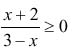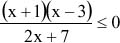Steps to solving quadratic or rational inequalities:

1.  Zero should be on one side of the inequality while everything else is on the other side.
2.  Factor whenever possible.
3.  Set the factors equal to zero and solve.
4.  Find a region or regions that satisfies the inequality. One way to do this is to graph the equation and then test values above or below the graph or axes.
5.  Check your answer – make sure that your endpoints do not make the original inequality undefined.

Practice

1.    3x2 - 11x - 4 < 0

2.    6x2 + 5x > 4

3.4.